# Probability

Probability defines the likelihood of occurrence of an event. There are many real-life situations in which we may have to predict the outcome of an event. We may be sure or not sure of the results of an event. In such cases, we say that there is a probability of this event to occur. It is to be known that probability and statistics are related to each other, where the first one deals with predicting future events and statistics deals with data handling, data representation.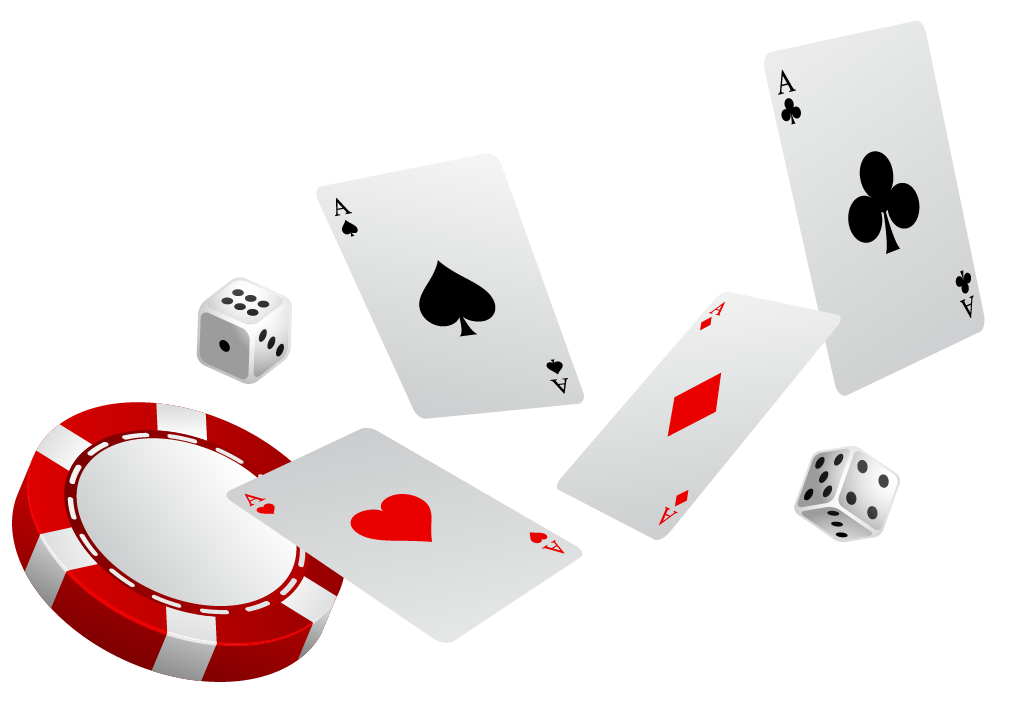In this mini-lesson, we will discuss about basics of probability, terms used in probability, probability formula, conditional probability, probability distribution, probability density function, law of total probability, Bayes' theorem.

## Lesson Plan

 1 What Is Probability? 2 Important Notes on Probability 3 Challenging Questions on Probability 4 Solved Examples on Probability 5 Interactive Questions on Probability

## Basics of Probability

Probability can be defined as the ratio of the number of favorable outcomes to the total number of outcomes of an event.

For example, let us consider the following statement.

Will it rain today?

The answer to this question is either "Yes" or "No." There is a likelihood to rain or not rain.

This is called probability.

Some important terms used in probability are as discussed below.

### Experiment

A trial or an operation conducted to produce an outcome is called an experiment.

### Sample Space

All the possible outcomes of an experiment together constitute a sample space. For example, the sample space of tossing a coin are head and tail.

### Favorable Outcome

An event that has produced the desired result or expected event is called a favorable outcome. For example, when we roll two dice, the possibility of getting number 4 is (1,3), (2,2), and (3,1).

### Trial

A trial denotes doing a random experiment.

### Random Experiment

An experiment in which we know all the possible outcomes but are not the exact income is called a random experiment. For example, when we toss a coin, we know that we would get a head or tail, but we are not sure of which one will appear.

### Event

The total number of outcomes of a random experiment is called an event.

### Equally Likely Events

Events that have the same chances or probability of occurring are called equally likely events. The outcome of one event is independent of the other.

For example, when we toss two coins, there is a chance that we get head while tossing both the coins.

### Exhaustive Events

When the set of all outcomes of an experiment is equal to the sample space, we call it an exhaustive event.

### Mutually Exclusive Events

Events that are not happening simultaneously are called mutually exclusive events. For example, the climate can be either hot or cold. We cannot experience the same weather simultaneously.

### Probability of an Event Formula

 $$Probability\: of\: an\: event\:P(E)\:$$=$$\: \dfrac{Number\: of\: favorable\: outcomes}{Sample Space}$$

## Tossing a Coin

Let us now look into the probability of tossing a coin and rolling a dice.

Paul and his friend Joe wanted to play a game. They both wanted to take the first turn to play.  Paul's mom asked Paul and John to decide on who wanted the head of the coin and who wanted the tail of the coin. Fortunately, they both decided on different options. John wanted a head and Paul wanted a tail. She then asked one of them to toss a coin and if head occurs John can take the first turn to play and if tail occurs Paul would take the first turn to play. They happily agreed on this method and enjoyed playing their game.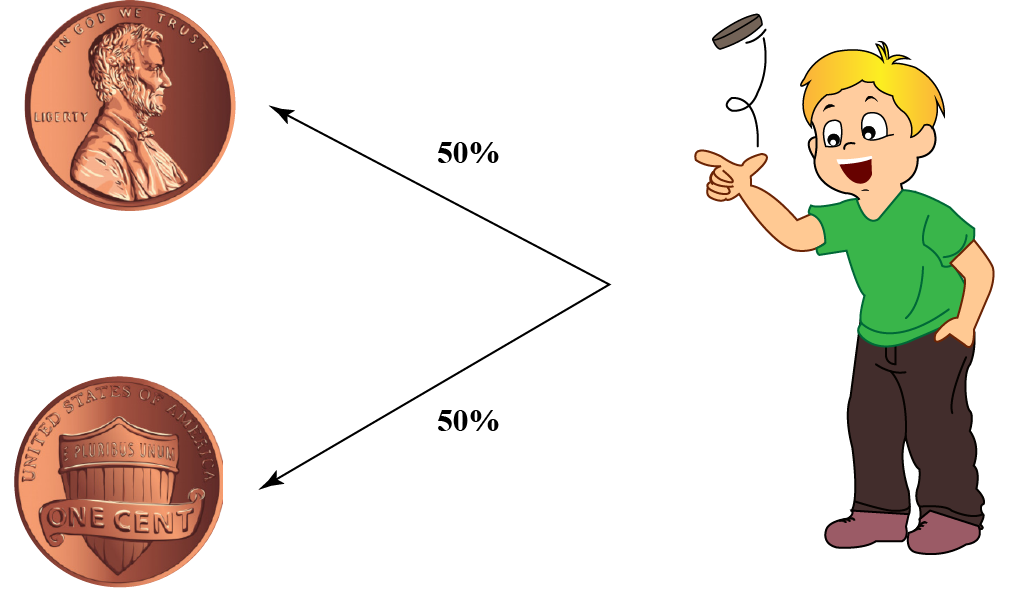We can also see coining tossing in games such as cricket to decide on who should bowl or bat first.

This method is used because the chances of getting a head and tail are equal.

This means there is a 50% chance of getting a head and a 50% chance of getting a tail.

### Tossing a Coin Probability Formula

While tossing a coin, there are two possible outcomes. One is head and the other is tail.

\begin{align} Total\: number\: of\: possible\: outcomes\: =\: 2\: (Head\: and\: Tail)\end{align}

\begin{align} Number\: of\: favorable\: outcome\: to\: get\: a\: head\:=\: 1\: (Head)\end{align}

\begin{align} Number\: of\: favorable\: outcome\: to\: get\: a\: tail\:=\: 1\: (Tail)\end{align}

Therefore,

\begin{align} Probability\: of \:getting\: a\: head\: =\: \dfrac{Number\: of\: favorable\: outcome\: to\: get\: a\: head}{Total\: number\: of\: possible\: outcomes} \end{align}

\begin{align} Probability\: of \:getting\: a\: tail\: =\: \dfrac{Number\: of\: favorable\: outcome\: to\: get\: a\: tail}{Total\: number\: of\: possible\: outcomes} \end{align}

So, the probability of getting a head = \begin{align} \dfrac{1}{2} \end{align}

the probability of getting a tail = \begin{align} \dfrac{1}{2} \end{align}

Please note that when we toss two or more coins together the probability of getting a head or tail for each coin may differ.

## Rolling a Die

### Rolling a Single Die

Dice finds its use in playing a lot of board games to decide how many squares are we supposed to move. Similar to tossing a coin, rolling a die also has a probability.

The set of possible outcomes when we roll a die are \begin{align} \{{1,2,3,4,5,6}\} \end{align}. We may get any number on the face of a die when we roll it.

For example,

The probability of getting a desired number while rolling a die is,

\begin{align} Probability\: of \:getting\: the\: desired\:number \: =\: \dfrac{Number\: of\: favorable\: outcome\: to\: get\: the\:desired\:number}{Total\: number\: of\: possible\: outcomes} \end{align}

Probability of getting a 3 while rolling a die = \begin{align} \dfrac{1}{6} \end{align} (Since there is a probability to get number \begin{align} 3\end{align} once)

### Rolling Two Dice

Sometimes we roll two dice together.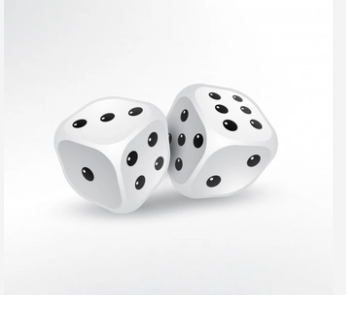All the probabilities of rolling two dice together are presented in the table below.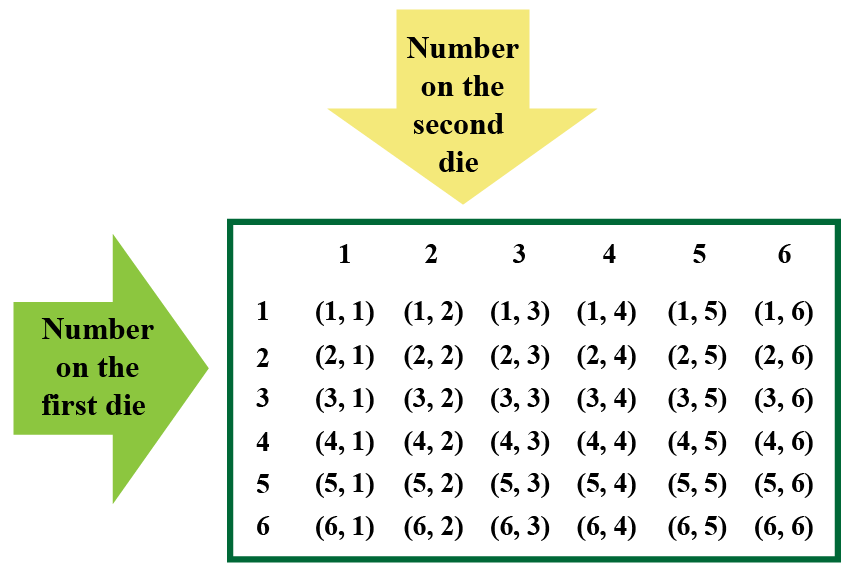## Rolling Three Or More Dice

As we see, when we roll a single die, there are 6 possibilities. When we roll two dice, there are 36 possibilities. When we roll 3 dice we get 216 possibilities. So a general formula to represent this is,

 \begin{align} Probability\: of\: rolling\: a\:die\: 'n' \: times\: =\: 6^n\end{align}

## Probability of Drawing Cards

A deck containing 52 cards are grouped into four suits of clubs, diamonds, hearts, and spades. Now let us discuss the probability of drawing cards from a pack.

The symbols on the cards are shown below. Spades and clubs are black cards. Hearts and diamonds are red cards.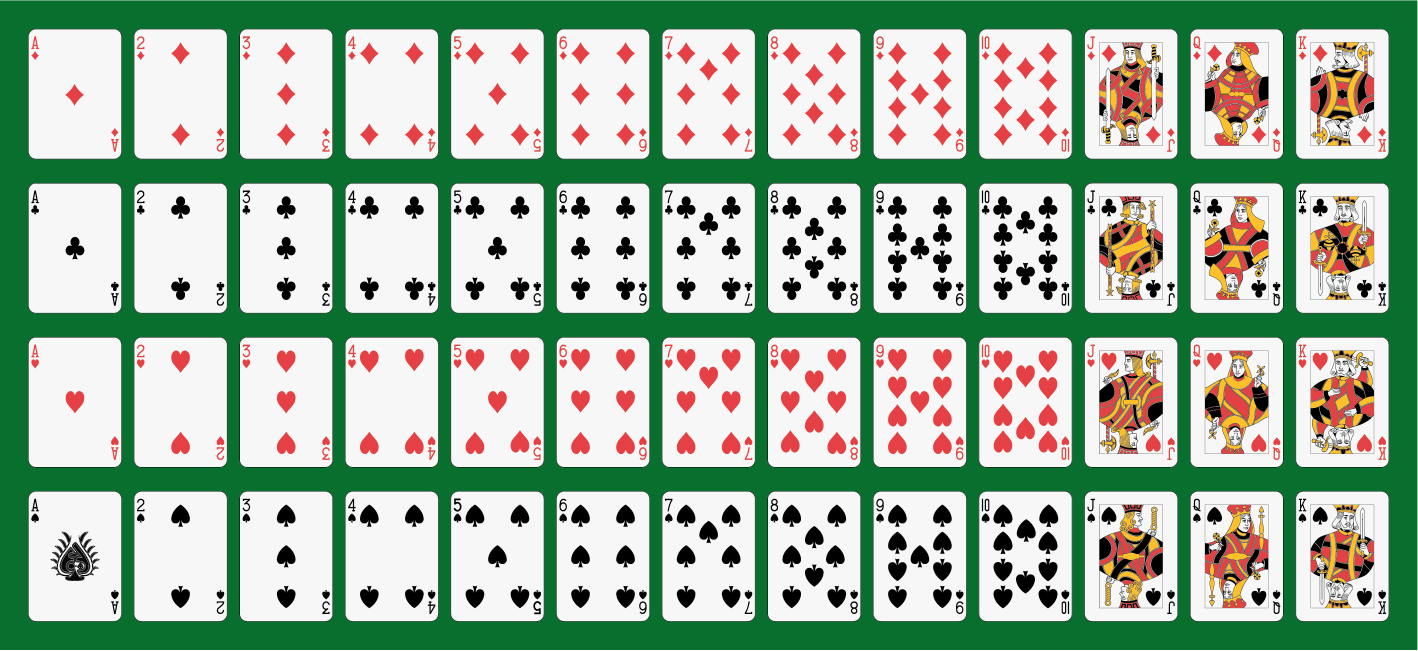In each suit there is an ace, king, queen, jack, 10, 9, 8, 7, 6, 5, 4, 3, 2. King, queen, and jack are called face cards. We can apply the same formula of probability to find the probability of drawing a single card or two or more cards.

For example, the probability of drawing a ace spade from a pack of 52 cards is  \begin{align}\dfrac{1}{52}\end{align}.

We will look into more examples in the Solved Examples section.

## Basic Theorems on Probability

Before getting to the theorems of probability, let us discuss a few important points.

The probability value ranges from 0 to 1, where 0 refers to the possibility of occurrence of an event and 1 denotes impossibility.

The sum of probability of all possible events is 1

### Theorem 1

\begin{align}P(A) = P(1-A')\end{align}

### Theorem 2

\begin{align}P(\phi) =0\end{align}

### Theorem 3

If there are two events A and B and A is a subset of B,  then,

\begin{align}P(A) \leq P(B)\end{align}

### Theorem 4

The probability of any event is always less than or equal to 1

\begin{align}P(A) \leq 1\end{align}

### Theorem 5

If there are two events A and B, then

\begin{align}P(A\cup B) = P(A) + P(B) - P(A\cap B)\end{align}

### Conditional Probability or Bayes' Theorem

Bayes' theorem describes the probability of an event based on the condition of occurrence of other events. It is also called conditional probability.

For example, let us assume that there are three bags with each bag containing some blue, green, and yellow balls. What is the probability of picking a yellow ball from the third bag? Since there are also blue and green coloured balls, we can arrive at the probability based on these conditions also. Such a probability is called a conditional probability.

The formula for Bayes' theorem is

 \begin{align}P(A|B) = \dfrac{P(A) P(B|A)} {P(B)}\end{align}

where \begin{align}P(A|B) \end{align} denotes how often event A happens on a condition that B happens.

where \begin{align}P(B|A) \end{align} denotes how often event B happens on a condition that A happens.

\begin{align}P(A) \end{align} the likelihood of occurrence of event A.

\begin{align}P(B) \end{align} the likelihood of occurrence of event B.

### Law of Total Probability

The Law of total probability states that when there is not enough information to find the probability of event A, then we take a related event B and use it to calculate the probability of A.

For example, consider the figure below.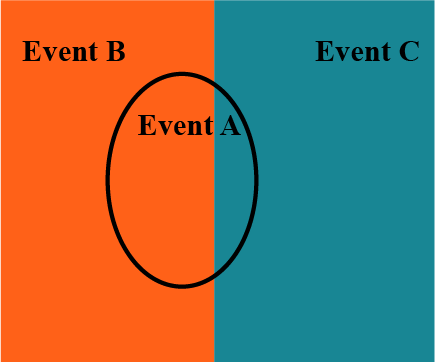In the figure, it is clear that the probability of A cannot be found alone. The probability of A depends on the occurrence of events  B and C. But it can be found collectively with the occurrence of condition B and condition C.

The formula for law of probability is

 \begin{align}P(A) = \sum_{n} \: P(A \cap B_n) \end{align}

Where,

\begin{align}n \end{align} is the total number of events.

\begin{align}B_n \end{align} is the total number of distinct events.

Probability
grade 10 | Questions Set 1
Probability
Probability